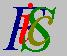Mauro Murzi's pages on Philosophy of Science - Quantum mechanics
Complex numbers

# 2. Mathematical elements.

Some mathematical instruments necessary for a comprehension of quantum mechanics are described: complex numbers, complex functions, derivatives and partial derivatives of complex functions.

## Complex numbers.

A complex number is a number of the type a+ib where a and b are real numbers, called respectively the real and the imaginary part, and i² = -1. If b=0 then the complex number is also a real number, that is a real number is a complex number which has no imaginary part.

Let z=a+ib be a complex number; from z others mathematical entities are defined:

• The complex conjugate of z is the complex number a-ib and is denoted by z*. If z is a real number then z=z*. Note that z·z*=a²+b² is a real number.
• The module (also called absolute value) of z is the real number √(a² + b²); it is denoted by | z |. Between the module and the complex conjugate holds the relation | z |² = z·z*.
• The square of z is the complex number z·z=a² - b² + 2abi and is denoted by z². In general, z² ≠ | z |². Only if z is a real number then z² = | z |².

The four basic operation (addition, subtraction, multiplication and division) between complex numbers are carried out like the corresponding operations on real polynomials, remembering that i² = -1. Some examples:

• (3+5i)(2-3i) = 6-9i+10i-15i² = 6+i+15 = 21+i
• (3-4i)+(6+i) = 3-4i+6+i = 9-3i
• (3-4i)-(6+i) = 3-4i-6-i = -3-5i